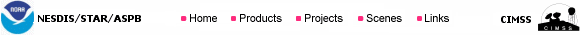## Ice Particle Habit Shortwave Optical Properties

#### Version 1.0

The shortwave optical properties of ice clouds for a variety of particle "habits" have been parameterized for use in radiative transfer models. The different habits are shown below. Click on the data link (above) to access the coefficients of the parameterization.A statistical relationship between the optical and microphysical properties of ice clouds with various particle habits was formulated as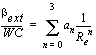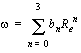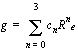where bext is the volume extinction coefficient, WC is the ice water content , w is the single scattering albedo, and g is the asymmetry parameter. The coefficients an, bn, and cn, are determined in a least squares sense with nonlinear regression. A different set of coefficients is obtained for every spectral band and every habit. The parameterization uses an effective "radius" defined by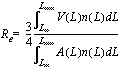where L is the maximum dimension of an ice crystal, V is the volume of the crystal, A is the projected area, and n(L) is a size distribution. The figure below shows the relationship between the effective radius and maximum dimension for 30 different size distributions.The asymmetry parameter is commonly used with the Henyey-Greenstein (HG) function as an approximation to the scattering phase function. The HG function is defined as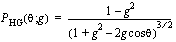The function represents forward scattering reasonably well but it does not capture backscattering behavior. This problem can be remedied through the use of the double Henyey-Greenstein function: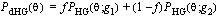where g2 can be assigned a negative value to account for a backscattering peak and f is a positive fraction in the range [0,1].

### References

Key, J., P. Yang, B. Baum, and S. Nasiri, 2002, Parameterization of shortwave ice cloud optical properties for various particle habits, J. Geophys. Res., 107(D13), 4181, doi:10.1029/2001JD000742. Electronic (PDF) version as published (copyright American Geophysical Union)